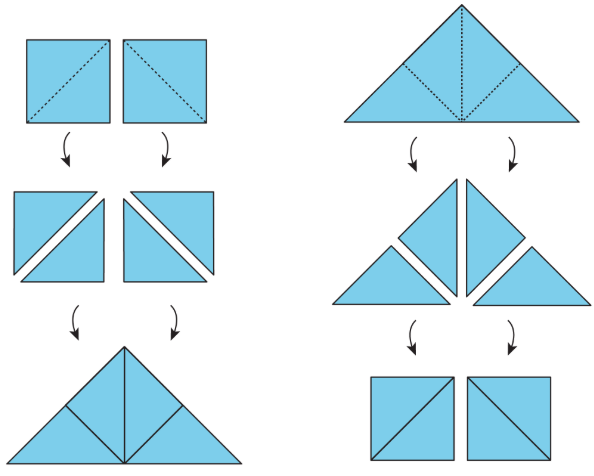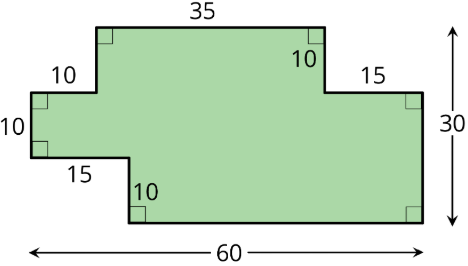$$\newcommand{\id}{\mathrm{id}}$$ $$\newcommand{\Span}{\mathrm{span}}$$ $$\newcommand{\kernel}{\mathrm{null}\,}$$ $$\newcommand{\range}{\mathrm{range}\,}$$ $$\newcommand{\RealPart}{\mathrm{Re}}$$ $$\newcommand{\ImaginaryPart}{\mathrm{Im}}$$ $$\newcommand{\Argument}{\mathrm{Arg}}$$ $$\newcommand{\norm}{\| #1 \|}$$ $$\newcommand{\inner}{\langle #1, #2 \rangle}$$ $$\newcommand{\Span}{\mathrm{span}}$$

# 1.1.2: Finding Area by Decomposing and Rearranging

•• Contributed by Illustrative Mathematics
• OpenUp Resources
$$\newcommand{\vecs}{\overset { \rightharpoonup} {\mathbf{#1}} }$$ $$\newcommand{\vecd}{\overset{-\!-\!\rightharpoonup}{\vphantom{a}\smash {#1}}}$$$$\newcommand{\id}{\mathrm{id}}$$ $$\newcommand{\Span}{\mathrm{span}}$$ $$\newcommand{\kernel}{\mathrm{null}\,}$$ $$\newcommand{\range}{\mathrm{range}\,}$$ $$\newcommand{\RealPart}{\mathrm{Re}}$$ $$\newcommand{\ImaginaryPart}{\mathrm{Im}}$$ $$\newcommand{\Argument}{\mathrm{Arg}}$$ $$\newcommand{\norm}{\| #1 \|}$$ $$\newcommand{\inner}{\langle #1, #2 \rangle}$$ $$\newcommand{\Span}{\mathrm{span}}$$ $$\newcommand{\id}{\mathrm{id}}$$ $$\newcommand{\Span}{\mathrm{span}}$$ $$\newcommand{\kernel}{\mathrm{null}\,}$$ $$\newcommand{\range}{\mathrm{range}\,}$$ $$\newcommand{\RealPart}{\mathrm{Re}}$$ $$\newcommand{\ImaginaryPart}{\mathrm{Im}}$$ $$\newcommand{\Argument}{\mathrm{Arg}}$$ $$\newcommand{\norm}{\| #1 \|}$$ $$\newcommand{\inner}{\langle #1, #2 \rangle}$$ $$\newcommand{\Span}{\mathrm{span}}$$

## Lesson

Let's create shapes and find their areas.

Exercise $$\PageIndex{1}$$: What is Area?

You may recall that the term area tells us something about the number of squares inside a two-dimensional shape.

1. Here are four drawings that each show squares inside a shape. Select all drawings whose squares could be used to find the area of the shape. Be prepared to explain your reasoning.Figure $$\PageIndex{1}$$: Four drawings that each show squares inside a shape. Shape A is broken up into large squares, Shape B is broken up into a combination of large and small squares, Shape C is broken up into a combination of large squares and white space, and Shape D is broken up into small squares.
1. Write a definition of area that includes all the information that you think is important.

Exercise $$\PageIndex{2}$$: Composing Shapes

This applet has one square and some small, medium, and large right triangles. The area of the square is 1 square unit.

Click on a shape and drag to move it. Grab the point at the vertex and drag to turn it.

1. Notice that you can put together two small triangles to make a square. What is the area of the square composed of two small triangles? Be prepared to explain your reasoning.
2. Use your shapes to create a new shape with an area of 1 square unit that is not a square. Draw your shape on paper and label it with its area.
3. Use your shapes to create a new shape with an area of 2 square units. Draw your shape and label it with its area.
4. Use your shapes to create a different shape with an area of 2 square units. Draw your shape and label it with its area.
5. Use your shapes to create a new shape with an area of 4 square units. Draw your shape and label it with its area.

Find a way to use all of your pieces to compose a single large square. What is the area of this large square?

Exercise $$\PageIndex{3}$$: Tangram Triangles

Recall that the area of the square you saw earlier is 1 square unit. Complete each statement and explain your reasoning.

1. The area of the small triangle is ____________ square units. I know this because . . .
2. The area of the medium triangle is ____________ square units. I know this because . . .
3. The area of the large triangle is ____________ square units. I know this because . . .

### Summary

Here are two important principles for finding area:

1. If two figures can be placed one on top of the other so that they match up exactly, then they have the same area.
2. We can decompose a figure (break a figure into pieces) and rearrange the pieces (move the pieces around) to find its area.

Here are illustrations of the two principles.Figure $$\PageIndex{2}$$: An image of two squares, which are then decomposed into four triangles, which are then rearranged into one large triangle. Another image of a large triangle that is decomposed into four smaller triangles, and then rearranged into two squares.
• Each square on the left can be decomposed into 2 triangles. These triangles can be rearranged into a large triangle. So the large triangle has the same area as the 2 squares.
• Similarly, the large triangle on the right can be decomposed into 4 equal triangles. The triangles can be rearranged to form 2 squares. If each square has an area of 1 square unit, then the area of the large triangle is 2 square units. We also can say that each small triangle has an area of $$\frac{1}{2}$$ square unit.

### Glossary Entries

Definition: Area

Area is the number of square units that cover a two-dimensional region, without any gaps or overlaps.

For example, the area of region A is 8 square units. The area of the shaded region of B is $$\frac{1}{2}$$ square unit.Figure $$\PageIndex{3}$$

Definition: Compose

Compose means “put together.” We use the word compose to describe putting more than one figure together to make a new shape.Figure $$\PageIndex{4}$$

Definition: Decompose

Decompose means “take apart.” We use the word decompose to describe taking a figure apart to make more than one new shape.Figure $$\PageIndex{5}$$

Definition: Region

A region is the space inside of a shape. Some examples of two-dimensional regions are inside a circle or inside a polygon. Some examples of three-dimensional regions are the inside of a cube or the inside of a sphere.

## Practice

Exercise $$\PageIndex{4}$$

The diagonal of a rectangle is shown.Figure $$\PageIndex{6}$$
1. Decompose the rectangle along the diagonal, and recompose the two pieces to make a different shape.
2. How does the area of this new shape compare to the area of the original rectangle? Explain how you know.

Exercise $$\PageIndex{5}$$

Priya decomposed a square into 16 smaller, equal-size squares and then cut out 4 of the small squares and attached them around the outside of original square to make a new figure.

How does the area of her new figure compare with that of the original square?Figure $$\PageIndex{7}$$
1. The area of the new figure is greater.
2. The two figures have the same area.
3. The area of the original square is greater.
4. We don’t know because neither the side length nor the area of the original square is known.

Exercise $$\PageIndex{6}$$

The area of the square is 1 square unit. Two small triangles can be put together to make a square or to make a medium triangle.Figure $$\PageIndex{8}$$

Which figure also has an area of $$1\frac{1}{2}$$ square units? Select all that apply.Figure A is composed of three small triangles, figure B is composed of three small triangles in a different arrangement, figure C is composed of one medium triangle and one small triangle, and figure D is composed of two small triangles and one square.
1. Figure A
2. Figure B
3. Figure C
4. Figure D

Exercise $$\PageIndex{7}$$

The area of a rectangular playground is 78 square meters. If the length of the playground is 13 meters, what is its width?

(From Unit 1.1.1)

Exercise $$\PageIndex{8}$$

A student said, “We can’t find the area of the shaded region because the shape has many different measurements, instead of just a length and a width that we could multiply.”Figure $$\PageIndex{10}$$: A multi-sided figure. The sides on top measure 10 units, 35 units, and 15 units. Two of the three sides on the left measure 10 units. One of the two sides on the right measures 10 units. One of the two sides on the bottom measure 15 units. The total width of the figure is 60 units, and the total height is 30 units. All angles are right angles.

Explain why the student's statement about area is incorrect.

(From Unit 1.1.1)